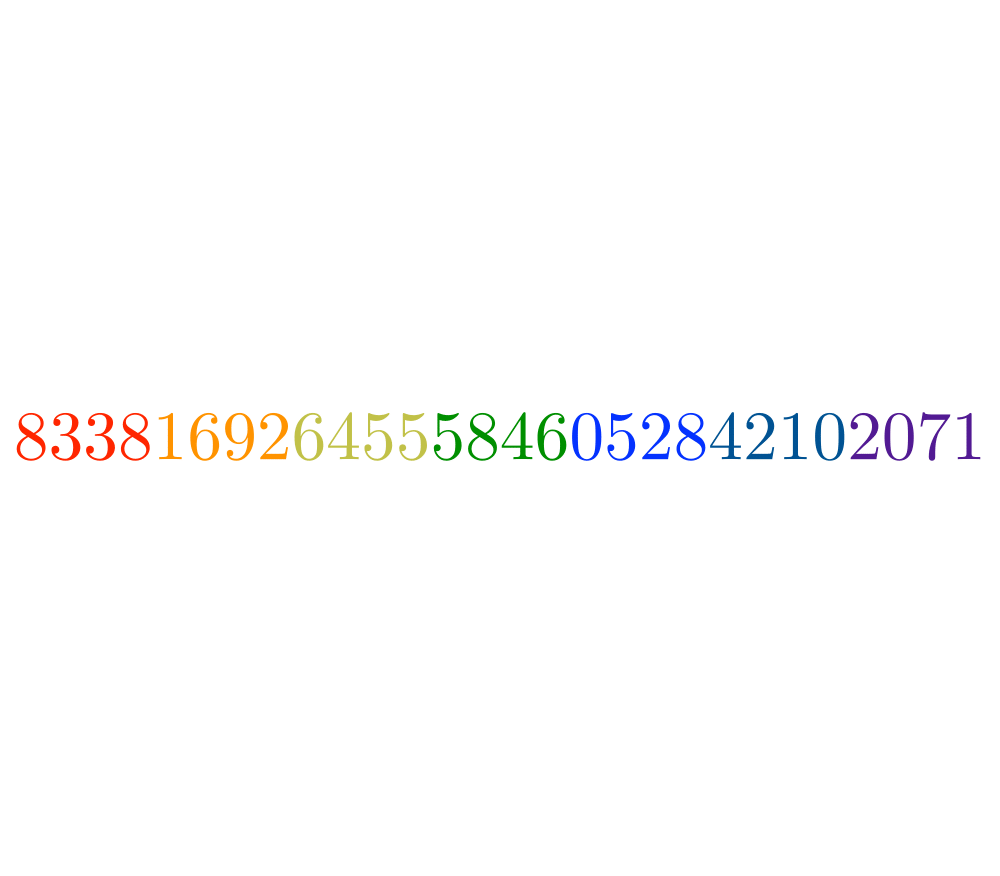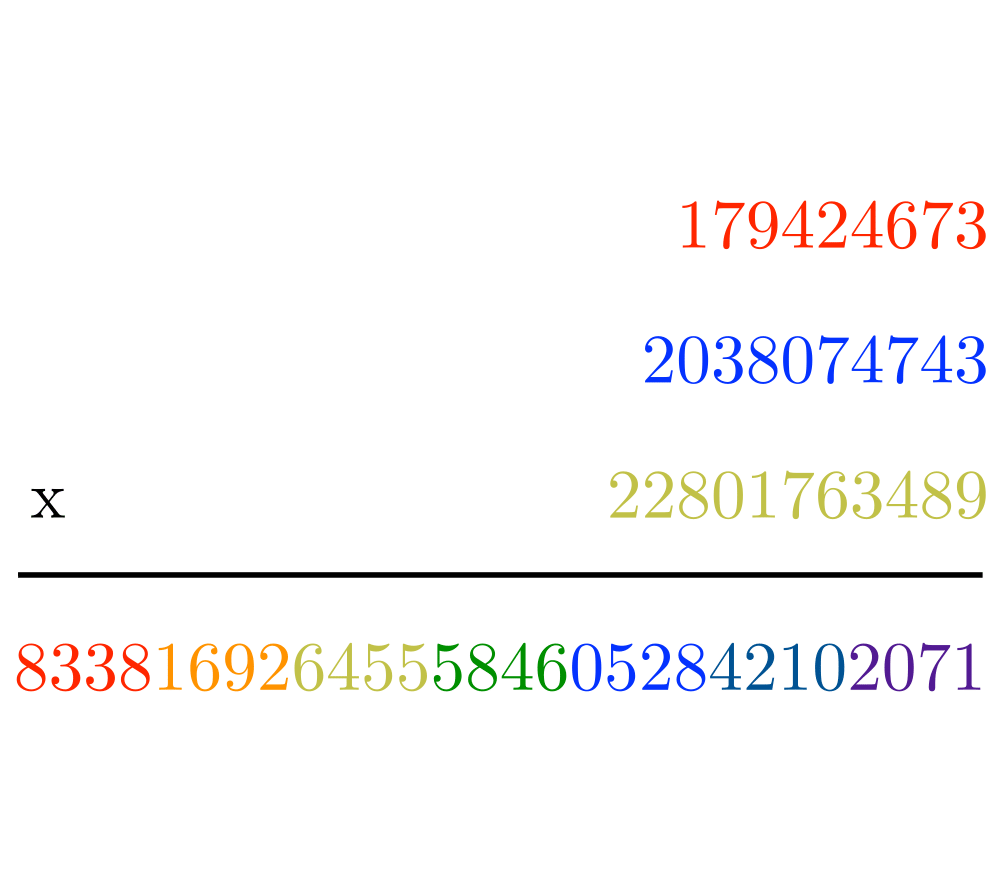# Factoring and Primality TestingInputOutput

Input Description: An integer $$n$$.
Problem: Is $$n$$ a prime number, and if not what are the factors of $$n$$?

Excerpt from The Algorithm Design Manual: Although factoring and primality testing are related problems, algorithmically they are quite different. There exist algorithms that can demonstrate that an integer is composite (i.e. not prime) without actually giving the factors. To convince yourself of the plausibility of this, note that you can demonstrate the compositeness of any nontrivial integer whose last digit is 0, 2, 4, 5, 6, or 8 without doing the actual division.

The simplest algorithm for both of these problems is brute-force trial division. To factor $$n$$, compute the remainder of $$n/i$$ for all $$1 < i \leq \sqrt{n}$$. The prime factorization of $$n$$ will contain at least one instance of every $$i$$ such that $$n/i = \lfloor n/i \rfloor$$, unless $$n$$ is prime. Make sure you handle the multiplicities correctly and account for any primes larger than $$\sqrt{n}$$.

Considerably faster factoring algorithms exist, whose correctness depends upon more substantial number theory. The fastest known algorithm, the number field sieve, uses randomness to construct a system of congruences, the solution of which usually gives a factor of the integer. Integers with as many at 128 digits have been factored using this method, although such feats require enormous amounts of computation.

Go To Main Page